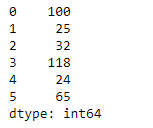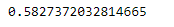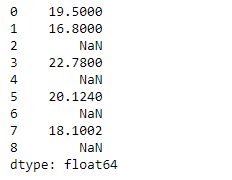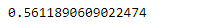Related Articles

# Python | Pandas Series.skew()

• Last Updated : 05 Feb, 2019

Pandas series is a One-dimensional ndarray with axis labels. The labels need not be unique but must be a hashable type. The object supports both integer- and label-based indexing and provides a host of methods for performing operations involving the index.

Pandas` Series.skew()` function return unbiased skew over requested axis Normalized by N-1. Skewness is asymmetry in a statistical distribution, in which the curve appears distorted or skewed either to the left or to the right.

Syntax: Series.skew(axis=None, skipna=None, level=None, numeric_only=None, **kwargs)

Parameter :
axis : Axis for the function to be applied on.
skipna : Exclude NA/null values when computing the result.
level : If the axis is a MultiIndex (hierarchical), count along a particular level, collapsing into a scalar.
numeric_only : Include only float, int, boolean columns.
**kwargs : Additional keyword arguments to be passed to the function.

Returns : skew : scalar or Series (if level specified)

Example #1 : Use `Series.skew()` function to find the skewness in the data of the given Series object.

 `# importing pandas as pd``import` `pandas as pd`` ` `# Creating the Series``sr ``=` `pd.Series([``100``, ``25``, ``32``, ``118``, ``24``, ``65``])`` ` `# Print the series``print``(sr)`

Output :Now we will use `Series.skew()` function to find the skewness in the data.

 `# find skewness``sr.skew()`

Output :As we can see in the output, `Series.skew()` function has successfully calculated the skewness in the data of the given Series object.

Example #2 : Use `Series.skew()` function to find the skewness in the data of the given Series object. We have some missing values in our series object, so skip those missing values.

 `# importing pandas as pd``import` `pandas as pd`` ` `# Creating the Series``sr ``=` `pd.Series([``19.5``, ``16.8``, ``None``, ``22.78``, ``None``, ``20.124``, ``None``, ``18.1002``, ``None``])`` ` `# Print the series``print``(sr)`

Output :Now we will use `Series.skew()` function to find the skewness in the data.

 `# find skewness``sr.skew(skipna ``=` `True``)`

Output :As we can see in the output, `Series.skew()` function has successfully calculated the skewness in the data of the given Series object. Missing values has been skipped while calculating the skewness in the given data.

Attention geek! Strengthen your foundations with the Python Programming Foundation Course and learn the basics.

To begin with, your interview preparations Enhance your Data Structures concepts with the Python DS Course. And to begin with your Machine Learning Journey, join the Machine Learning – Basic Level Course

My Personal Notes arrow_drop_up